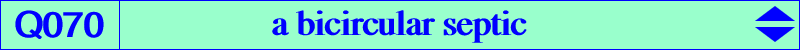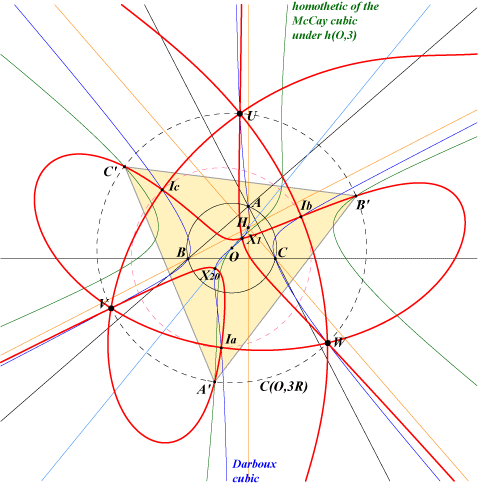too complicated to be written here. Click on the link to download a text file.X(1), X(20) excenters other points belowIn general, there is only one point Q whose polar conic in a cubic is a circle and this point Q does not lie on the cubic. When the cubic is an isogonal pK with pivot P, Q070 is the locus of P such that there is at least one point Q on the cubic having a circular polar conic. Q070 is a bicircular septic having three real asymptotes which are the altitudes of ABC. Q070 contains : – the in/excenters which are nodes on the curve. The nodal tangents are parallel to the asymptotes of the Feuerbach hyperbola for the incenter and the Boutin hyperbolas for the excenters. – three triple points U, V, W also lying on the Darboux cubic, on K077, on the circle C(O, 3R), on three hyperbolas described in the Darboux cubic page. – three other points A', B', C' on this same circle and on the homothetic of the McCay cubic under h(O,3). Hence, they are the vertices of an equilateral triangle. – the de Longchamps point X(20). The corresponding cubic is the Darboux cubic and Q is the circumcenter O. The polar conic is not a proper circle since it decomposes into the line at infinity and the inflexional tangent at O.